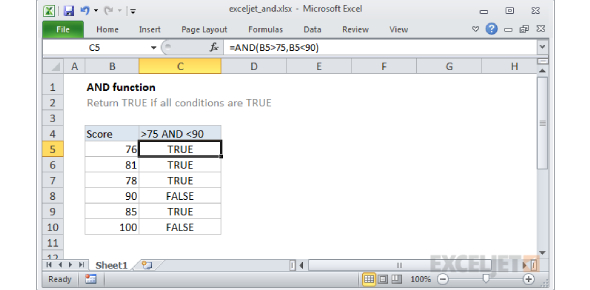# MS Excel Formulas And Functions Trivia Quiz!

26 Questions | Total Attempts: 115Settings.

• 1.
• A.

=sumif

• B.

=sum

• C.

=

• D.

=sumif(

• 2.
Common Functions that we use most often are:
• A.

All of the above

• B.

=Sum

• C.

=Average

• D.

=Min

• E.

=Max

• 3.
In "=SUM(number1 [,number2 ,number3 ...])" number 1, number 2, and number 3 may be numbers or __________.
• A.

Symbols

• B.

Symbols fact

• C.

Cell references

• D.

Cell cover

• 4.
The divide symbol is
• A.

DD

• B.

D

• C.

\

• D.

/

• 5.
Ctrl+B key makes the shortcut for making font in selected cell bold.
• A.

True

• B.

False

• 6.
A function inside another function is called a _____________ function.
• A.

Complex

• B.

Nested

• C.

All of the above

• D.

None of the above

• 7.
Microsoft Excel 2013 is a ___________________
• A.

Calculation package

• B.

Word processing package

• C.

• D.

Mathematics package

• 8.
Which of the following methods allows you to insert an Image into your Excel spreadsheet?
• A.

Go to the insert tab on the ribbon and select object. Choose Image from the box and select Ok

• B.

Simply drag and drop your Adobe Photoshop image file directly onto the worksheet you want to add it to

• C.

Microsoft Office is not compatible with image products

• D.

Select Open from the Office Button. Browse to your image file, select the checkbox labeled Insert into current document and hit open.

• 9.
To name a cell or range, you use the _______________
• A.

Format cell

• B.

Names box

• C.

Define name

• D.

Paste name

• 10.
As you begin to type a function name within a formula into a cell, a list of functions that begin with the letters you typed appears.
• A.

True

• B.

False

• 11.
An Excel spreadsheet is also called a _______________________ .
• A.

Workbook

• B.

Cell calculator

• C.

Worksheet

• D.

VB

• 12.
When using a databar, changing the value of one cell in the range will not affect the barsize of all the other cells.
• A.

True

• B.

False

• 13.
You can press ___________ to move between open Workbook
• A.

Shift + F6

• B.

F6

• C.

Control + F6

• D.

Control + Page down

• 14.
The function arguments dialog box tells you how to use functions.
• A.

True

• B.

False

• 15.
The function arguments dialog box tells you how to use functions.
• A.

Hold down the ALT key

• B.

Hold down the SHIFT key

• C.

Hold down the INSERT key

• D.

Hold down the CTRL key

• 16.
When a formula is written in a cell. It appears in the the active cell in which it is being written and in the __________________ bar as well.
• A.

Name Box

• B.

• C.

Formula

• D.

Title bar

• 17.
Which of the following functions is correctly written?
• A.

=IF(B2>50000,"rich","poor")

• B.

IF(B2>50000,rich,poor)

• C.

=IF(B2>50000),"rich","poor"))

• D.

=IF(B2>50000."rich","poor")

• 18.
Rows are identified by a _______________________
• A.

Column

• B.

Formula

• C.

Cell

• D.

Number

• 19.
To sort data in an Excel worksheet you can use the ______.
• A.

Sort command on the Formulas tab

• B.

Sort A to Z and Sort Z to A buttons

• C.

Quicksort Options

• D.

Any of the above

• 20.
All forumulas in Excel begin with the following symbol
• A.

+

• B.

-

• C.

(

• D.

=

• 21.
Which of the following option is a formula?
• A.

A1 = A5

• B.

=SUM=(A1:A5))

• C.

=SUM(A1:A5)

• D.

=(A1 = A5)

• 22.
A selection of multiple cells is called a
• A.

Range

• B.

Group

• C.

Column

• D.

Row

• 23.
In which view can you see the header and footer areas of a worksheet .
• A.

Page layout view

• B.

Page break preview

• C.

Normal view

• D.

Preview

• 24.
What is the function of the word '=SUM' at the beginning of an Excel spreadsheet formula?
• A.

To inform the computer that an arithmetic function will occur

• B.

To tell the person viewing that this is a function and it should be added together

• C.

• D.

To calculate all the data correctly without any mistakes

• 25.
An Excel spreadsheet is primarily used for calculating which of the following options.
• A.

Finances

• B.

Data

• C.

Numbers

• D.

All of the above

Related TopicsBack to top